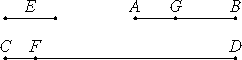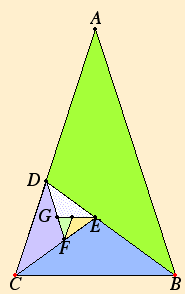# Proposition 2

If, when the less of two unequal magnitudes is continually subtracted in turn from the greater that which is left never measures the one before it, then the two magnitudes are incommensurable.

There being two unequal magnitudes AB and CD, with AB being the less, when the less is continually subtracted in turn from the greater, let that which is left over never measure the one before it.

I say that the magnitudes AB and CD are incommensurable.

If they are commensurable, then some magnitude E measures them.Let AB, measuring FD, leave CF less than itself, let CF measuring BG, leave AG less than itself, and let this process be repeated continually, until there is left some magnitude which is less than E.

Suppose this done, and let there be left AG less than E.

Then, since E measures AB, while AB measures DF, therefore E also measures FD. But it measures the whole CD also, therefore it also measures the remainder CF. But CF measures BG, therefore E also measures BG. But it measures the whole AB also, therefore it also measures the remainder AG, the greater the less, which is impossible.

X.Def.I.1

Therefore no magnitude measures the magnitudes AB and CD. Therefore the magnitudes AB and CD are incommensurable.

Therefore, if, when the less of two unequal magnitudes is continually subtracted in turn from the greater that which is left never measures the one before it, then the two magnitudes are incommensurable.

Q.E.D.

## Guide

Antenaresis (also called the Euclidean algorithm), first used in proposition VII.1, is again used in this proposition. Beginning with two magnitudes, the smaller, whichever it is, is repeated subtracted from the larger. Proposition VII.1 concerns relatively prime numbers. It is similar to this proposition, but its conclusion is different.

Heath claims that Euclid uses X.1 to prove this proposition, in particular, to show that antenaresis eventually leaves some magnitude which is less than E. It is hard to tell what Euclid thought his justification was. Since both magnitudes are multiples of E, whatever justification Euclid intended back in proposition VII.2 works just as well here. Euclid did, however, put X.1 just before this proposition, perhaps for an intended logical connection. If so, there is a missing statement to the effect that GB is greater than half of AB, and so forth, so that X.1 might be invoked.

#### An example of incommensurable magnitudes

 Consider the 36°-72°-72° triangle constructed ABC in proposition IV.10. This triangle was used in the following proposition IV.11 to construct regular pentagons. When its base BC is subtracted from a side AC then the remainder CD is the base of a similar triangle BCD. Likewise, when the base CD of this new triangle is subtracted from its side BD then the remainder DE is the base of yet another smaller similar triangle CDE. And so forth. Thus, when we begin with the two lines AB and BC and apply the algorithm of antenaresis to them, we get a series of lines which never ends AB, BC, CD, DE, EF, and so forth, and these lines form a never-ending continued proportion. AB : BC = BC : CD = CD : DE = DE : EF = ... Thus, according to this proposition, the two quantities AB and BC are incommensurable. Cutting the line AB at C to make this ratio AB : BC is called in VI.Def.3 cutting AB into extreme and mean ratio. A more recent name for this ratio is the “golden ratio.”#### Use of this proposition

This proposition is used in the next one.